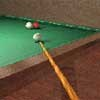#### You may also like### Squash

If the score is 8-8 do I have more chance of winning if the winner is the first to reach 9 points or the first to reach 10 points?### Snooker

A player has probability 0.4 of winning a single game. What is his probability of winning a 'best of 15 games' tournament?### Snooker Frames

It is believed that weaker snooker players have a better chance of winning matches over eleven frames (i.e. first to win 6 frames) than they do over fifteen frames. Is this true?

# Knock-out

##### Age 16 to 18 Challenge Level:

This solution was sent in by Paul from Berkhamsted Collegiate School.

To solve this problem we can, without loss of generality, think of the first player as being at the top of the draw. We MUST also assume that the chances of winning any given match is $1/2$. Then the chance of the players meeting in the first round (with $2^n$ participants) is $p=1/(2^{n} - 1)$.

The chances of the two players not meeting in the first round and winning their first round matches is: $$(1 - {1\over 2^{n} - 1})\times {1\over 4} = {2^{n-1}-1\over 2(2^n - 1)}.$$ It follows that the chance of the two players being drawn to meet in the second round is: $${2^{n-1}-1\over 2(2^n - 1)}\times {1\over(2^{n-1} - 1)} = {1\over 2(2^n-1)}= {p\over 2}.$$ So the odds of them meeting in the second round is half those of meeting in the first round. By the same reasoning the probability of meeting in each subsequent round is half the probability of meeting in the previous round.

For $2^n$ paricipants there will be $n$ rounds, so we can sum ALL the probabilities of meeting in a certain round, and we get \eqalign{ {1\over 2^n - 1} \left({1\over 1} + {1\over 2} + {1\over 4} + \cdots {1\over 2^{n-1}}\right) &= {1\over 2^n - 1} \left(2- {1\over 2^{n-1}}\right) \\ &= {1\over 2^n - 1}\left( {2^n - 1\over 2^{n-1}}\right) \\ &= {1\over 2^{n-1}}.} So the probability of the two players meeting if there are $2^n$ players competing is ${1\over 2^{n-1}}$.